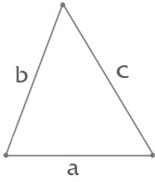Side a:   Side b:   Side c:Triangle is a polygon with three vertices, not lying on a one line, connected with three edges. Formula for perimeter of a triangle: P=a+b+c, where a, b, c - sides of a triangle